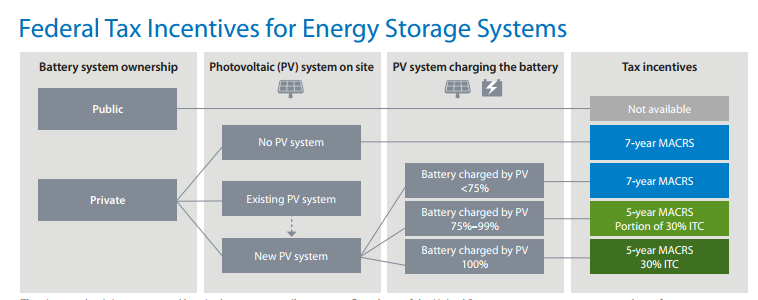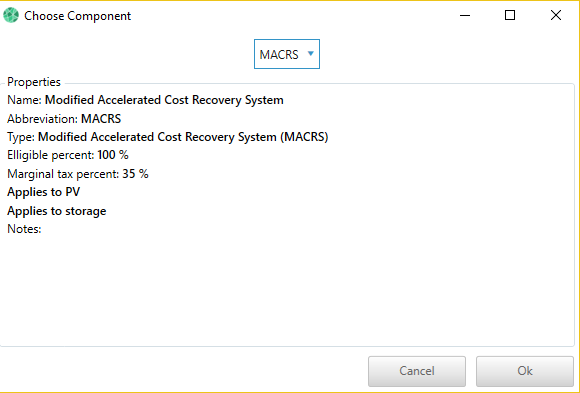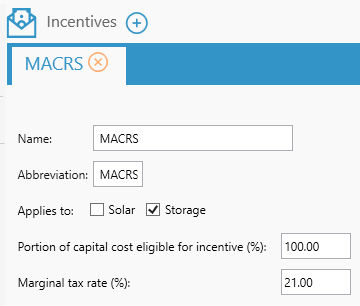# HOMER Grid 1.3

 Navigation: Design > Incentives Modified Accelerated Cost Recovery SystemModified Accelerated Cost Recovery System (MACRS) depreciation apply to storage depending on who owns the battery and how the battery is charged. The following schematic published by NREL explains which type of MACRS applies to which system:You can find the exact schedule for MACRS (Table A-1) in this IRS document: https://www.irs.gov/pub/irs-pdf/p946.pdfTable: MACRS Schedule by IRS

In HOMER Grid, you can apply MACRS to the following components:

Solar

StorageBelow is an explanation of the various inputs in MACRS

 Variable Description Marginal tax percent (%) The percentage of tax rate on the purchased asset Eligible percent (%) Percentage of the capital cost eligible for the incentive Applies to The components that this bonus depreciation applies to

## Example:

Let us assume a Storage of 100 kWh with a total capital cost of 100,000 \$. Let us apply the below MACRS incentive to the PV component:Let us assume that our simulation did not include a PV component. From the schematic from NREL, we know that this storage system is eligible for a 7 year MACRS. Then according to the 7 year MACRS schedule from IRS:

 Year Percentage (%) MACRS value (\$) 0 0.1429 0.1429 * 0.21 * 100,000 = 3001 1 0.2449 0.2449 * 0.21 * 100,000 = 5143 2 0.2449 0.2449 * 0.21 * 100,000 = 3673 3 0.1249 0.1249 * 0.21 * 100,000 = 2623 4 0.0893 0.0893 * 0.21 * 100,000 = 1875 5 0.0892 0.0892 * 0.21 * 100,000 = 1873 6 0.0893 0.0893 * 0.21 * 100,000 = 1875 7 0.0446 0.0446 * 0.21 * 100,000 = 937

The Homer Support site has a searchable knowledgebase and additional support options. HOMER Online contains the latest information on model updates, as well as sample files, resource data, and contact information. ©2017 HOMER Energy, LLC